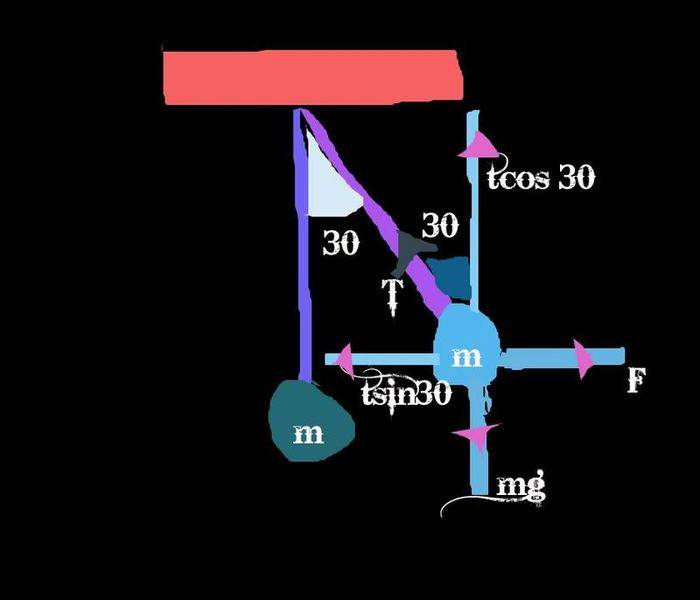# Tension/Force problem

• kxk010

#### kxk010

First time taking physics in many years, so please forgive the simplistic nature of this problem.

A 2.0 kg ball tied to a string fixed to the ceiling is pulled to one side by a force F. Just before the ball is released, (a) how large is the force F that is holding the ball in position and (b) what is the tension in the string?

Also given: the angle between the string and the ceiling is 30 degrees.

I understand everything up to the point that I've found the components of T and calculated:

sum of x forces = Tcos30 (since in eqb sum of x forces=0)
sum of y forces = Tsin30 (since in eqb sum of y forces =0)

In the solutions manual, they are then taking F/w (force vector in positive-x direction/ weight vector in negative-y direction) = Tcos30/Tsin30

I don't understand why they are dividing F by w. I see that this will eliminate T so that you can solve for F, however, the decision to divide F by w seems arbitrary. Either that or I'm fundamentally missing something. Any insight?

sum of x forces = Tcos30 (since in eqb sum of x forces=0)
sum of y forces = Tsin30 (since in eqb sum of y forces =0)
What you have are not the sum of forces, but just the x and y components of the tension.

What other forces act on the ball?
Write equations for ƩFx = 0 & ƩFy = 0.

(I presume that the given force F is horizontal?)

What you have are not the sum of forces, but just the x and y components of the tension.

What other forces act on the ball?
Write equations for ƩFx = 0 & ƩFy = 0.

(I presume that the given force F is horizontal?)

Yes, the force F is horizontal.

ƩFx = F - Tx = 0
so F = Tx
since Tx = Tsin30, F=Tsin30

ƩFy= Ty - W = 0
so Ty - w = 0
Ty = w
Tsin30 = w

So, in this instance, it turns out that the x and y components of the tension force are equal to F and W, respectively. So that's all I should be using to solve, right? Confused on the next step from here.

What we have here is thisnow all forces must cancel each other

so u get 2 equations and then solve for force

Tension can be found similarly

Tsin30 = mg...equation (1)
F= Tcos30...equation (2)

m is given so find tension using equation 1
then put that value in equation 2 to get force

Last edited:
Welcome to the forum kxk010!
You need to solve for F. The problem is of course that you don't know T so you have two unknowns.
You have to figure out a way to get rid of one of the two variables (T) .One way to go is to divide one by the other, as your solutions manual did, a substitution would also be fine.

Edit.

Welcome to the forum kxk010!
You need to solve for F. The problem is of course that you don't know T so you have two unknowns.
You have to figure out a way to get rid of one of the two variables (T) .One way to go is to divide one by the other, as your solutions manual did, a substitution would also be fine.

Ah, ok. So really you can take these 2 equations that you've "generated" and use them in any way necessary to isolate and solve for variables.

Yes, the force F is horizontal.

ƩFx = F - Tx = 0
so F = Tx
since Tx = Tsin30, F=Tsin30

ƩFy= Ty - W = 0
so Ty - w = 0
Ty = w
Tsin30 = w

So, in this instance, it turns out that the x and y components of the tension force are equal to F and W, respectively. So that's all I should be using to solve, right?
Is the 30 degrees the angle between the string and the ceiling, as you said before? If so, rethink those components--you have them reversed.
Confused on the next step from here.
Once you get those equations fixed, you just have to solve them simultaneously for the unknowns T & F. There are several ways to do that--take your pick. One way, like they show in your solutions manual, is to just divide the two equations by each other. That will eliminate T and allow you to get F. Try it and see.

Is the 30 degrees the angle between the string and the ceiling, as you said before? If so, rethink those components--you have them reversed.

Once you get those equations fixed, you just have to solve them simultaneously for the unknowns T & F. There are several ways to do that--take your pick. One way, like they show in your solutions manual, is to just divide the two equations by each other. That will eliminate T and allow you to get F. Try it and see.

You're right, I mistyped and F=Tcos30. Sorry about that. I seem to be stuck in this rigid mindset from other science classes that there is a sequential set of steps to solve every problem. Definitely finding that's not the case and there are multiple options (within limits) for the same problem. Thanks for all the help!